An introduction to Bayesian methods in ML

A two-day workshop introducing Bayesian modelling, using practical examples and probabilistic programming.

$$P(\theta | D) = \frac{P(D | \theta) P(\theta)}{P(D)} \hspace{1em} \text{Bayes’ rule}$$

There are two major philosophic interpretations of probability: frequentist and Bayesian. The frequentist interpretation is based on the idea that probability represents the long-term frequency of events. The Bayesian interpretation is based on the idea that probability represents the degree of belief in an event. The Bayesian interpretation can sometimes be more intuitive, and more naturally used to tackle certain problems.

The Bayesian approach is particularly well-suited for modeling uncertainty about a model’s parameters. This is because it allows for the use of prior knowledge to update their posterior probability distribution, which is not directly possible using the frequentist approach.

In this course we introduce the participants to Bayesian methods in machine learning. We start with a brief introduction to Bayesian probability and inference, then review the major approaches for the latter discussing their advantages and disadvantages. We introduce the model-based machine learning approach and discuss how to build probabilistic models from domain knowledge. We do this with the probabilistic programming framework pyro, working through a number of examples from scratch. Finally, we discuss how to criticise and iteratively improve a model.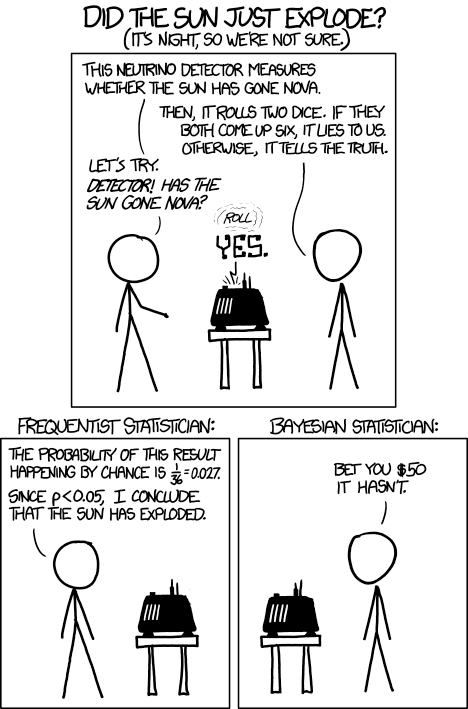XKCD 1132

# Learning outcomes

• Get to know the Bayesian methodology and how Bayesian inference can be used as a general purpose tool in machine learning.
• Understand computational challenges in Bayesian inference and how to overcome them.
• Get acquainted to the foundations of approximate bayesian inference.
• Take first steps in probabilistic programming with pyro.
• Get to know the model-based machine learning approach.
• Learn how to build probabilistic models from domain knowledge.
• Learn how to explore and iteratively improve your model.
• See many prototypical probabilistic model types, e.g. Gaussian mixture, Gaussian processes, latent Dirichet allocation, variational auto-encoder, or Bayesian neural networks.
• Learn how to combine probabilistic modelling with deep learning.

# Structure of the workshop

## Part 1: Introduction to Bayesian probability and inference

We start with a brief introduction to Bayesian probability and inference, then review the basic concepts of probability theory under the Bayesian interpretation, highlighting the differences to the frequentist approach.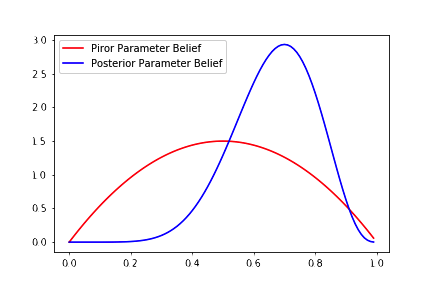Parameter learning in the Bayesian setup

• Recap on Bayesian probability.
• Example: determine the fairness of a coin.
• Introduction to pyro.

## Part 2: Exact inference with Gaussian processes

Gaussian processes are a powerful tool for modelling uncertainty in a wide range of problems. We give a brief introduction to inference with Gaussian distributions, followed by the Gaussian process model. We use the latter to model the CO2 data from the Mauna Loa observatory. Finally, we explain how sparse Gaussian processes allow working with larger data sets.Sparse Gaussian process posterior with $4$ pseudo-observations

• Inference with Gaussian variables.
• The Gaussian process model.
• Example: Manua Loa CO2 data.
• Sparse Gaussian processes.

## Part 3: Approximate inference with Markov chain Monte Carlo (MCMC)

Because computing the posterior distribution is often intractable, approximate techniques are required. One of the oldest and most popular approaches is Markov chain Monte Carlo (MCMC): Through a clever procedure it is possible to sample from the posterior rather than computing its analytic form. We start with a brief introduction the general class of methods, followed by the Metropolis-Hastings algorithm. We conclude with Hamiltonian MCMC and apply these algorithms to the problem of causal inference.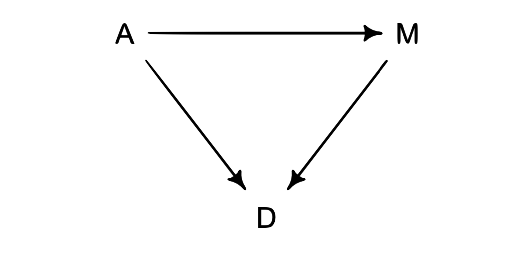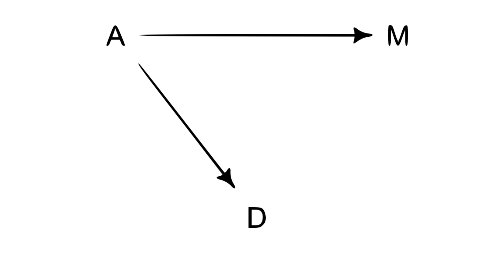Causal models for age (A), marriages (M), divorces (D)

• Introduction to MCMC.
• Example: King Markov and his island kingdom.
• Metropolis-Hastings, Hamiltonian MCMC, and NUTS.
• Example: Marriages, divorces and causality.

## Part 4: Approximate inference with stochastic variational inference (SVI)

MCMC methods suffer from the curse of dimensionality. In order to overcome this problem stochastic variational inference (SVI) can be used. SVI is a powerful tool for approximating the posterior distribution using a parametric family. After explaining the basics of SVI, we discuss common approaches to design parametric families, such as the mean field assumption. We demonstrate the power of SVI using variational auto-encoders to model semantic properties of yearbook images taken within the span of a full century.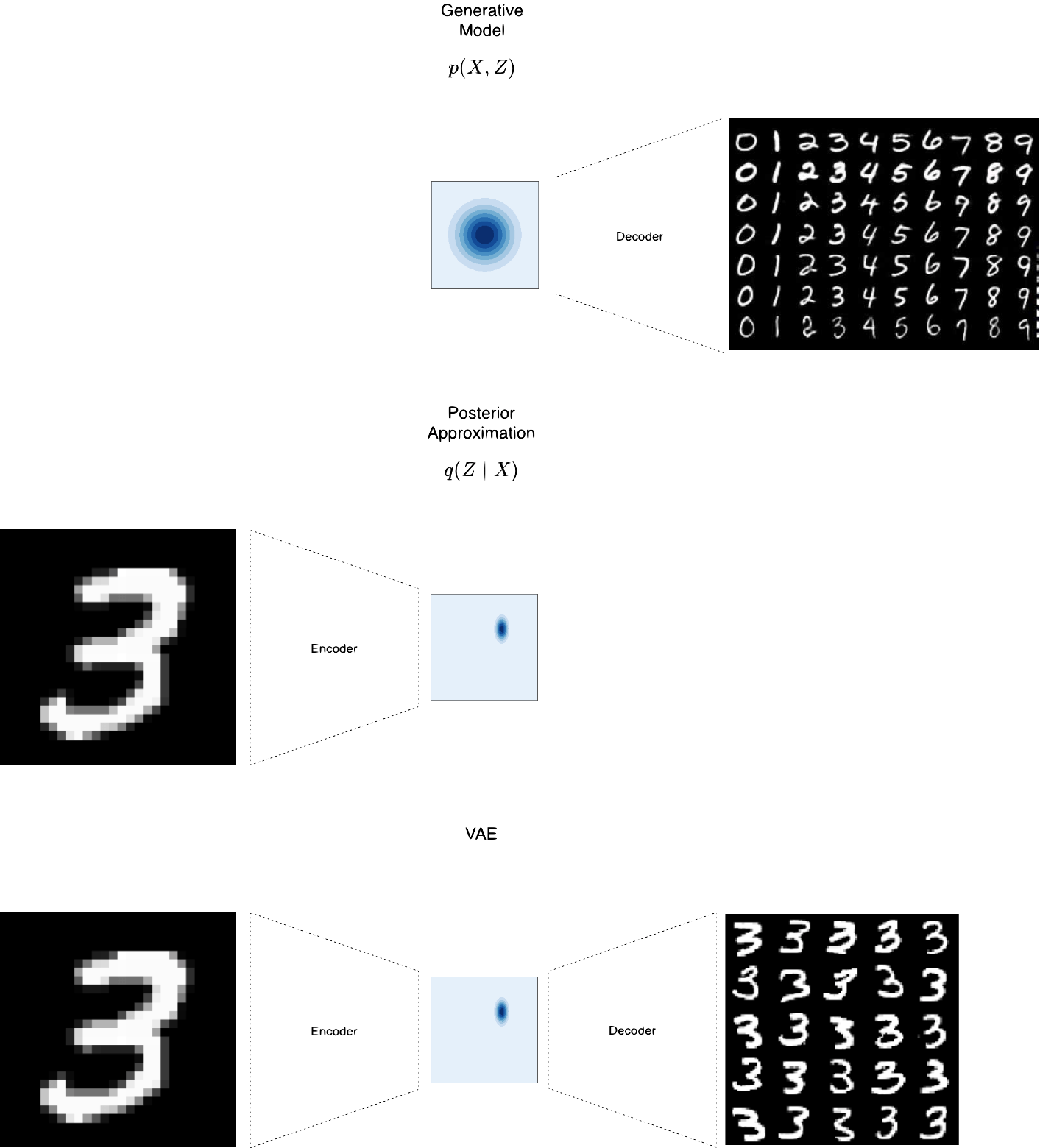Variational auto-encoder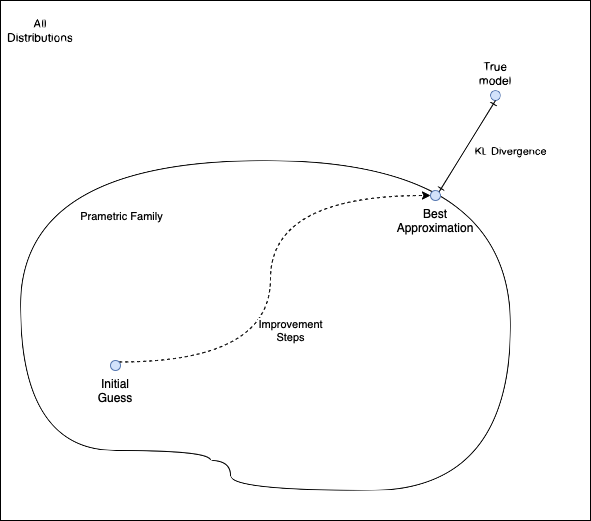Stochastic variational inference schema

• Introduction to SVI.
• Posterior approximations: the mean field assumption.
• Amortized SVI and variational auto-encoders.
• Example: Modeling yearbook faces through the ages with variational auto-encoders.

## Part 5: Laplace approximation and Bayesian neural networks

In this part we introduce the Laplace approximation and discuss how it can be used to approximate the posterior of the parameters of a neural network given the training data. The method combines a Gaussian distribution as the posterior approximation with a particular estimation technique. We then comment on how Bayesian neural networks can be used to obtain well-calibrated classifiers.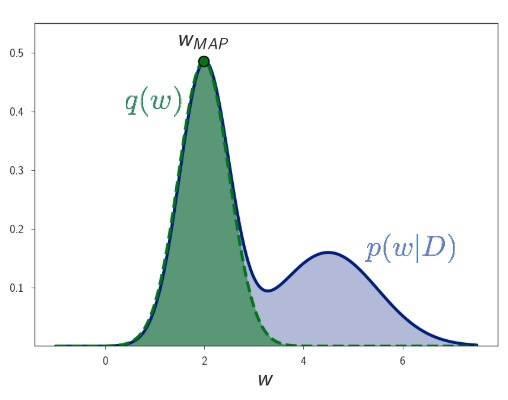Laplace Approximation

• Introduction to Laplace approximation.
• Bayesian neural networks and the calibration of classifiers.
• Example: Bayesian neural networks for wine connoisseurs.

The course contains a number of additional topics not included in the schedule. These are made available to the participants after the workshop, but, time allowing, we cover one or two of these topics chosen by the audience.

• Example: Understanding influence factors for the development of asthma through Markov chains.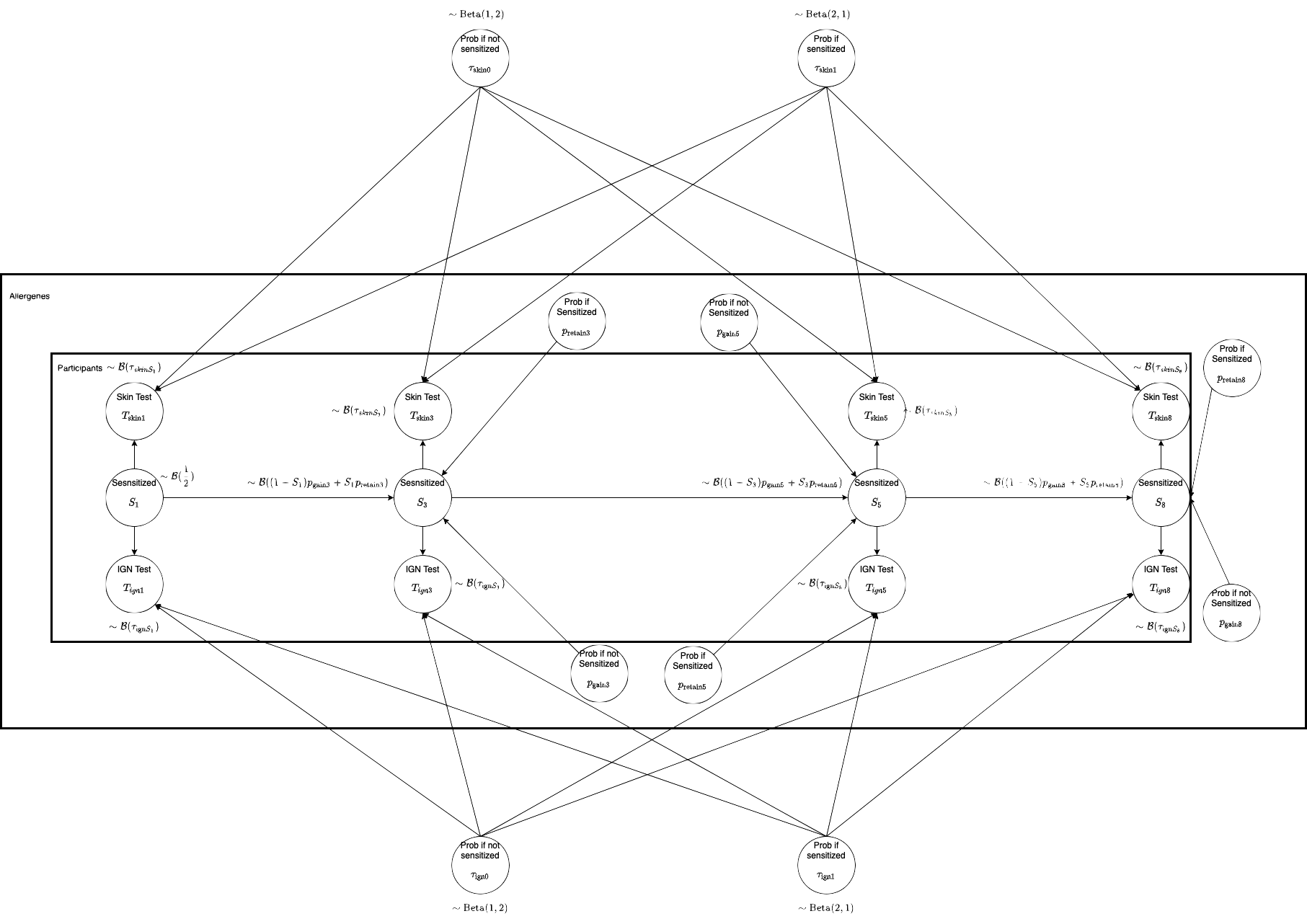Graphical model for the development of allergies
• Example: Satellite image clustering with mixture models and latent Dirichlet allocation like models.Local marginal distributions of the color channels in a satellite image
• Example: Assessing skills by questionnaire’s data with latent skill models.

# Prerequisites

• Probabilistic modelling demands a good understanding of probability theory and mathematical modelling. The participants should be familiar with the basic concepts.
• We assume prior exposure to machine learning and deep learning. We will not cover these in the course.
• Knowledge in python is required in order to complete the exercises. Experience with pytorch is not required but can be helpful.

# Companion seminar

Accompanying the course, we offer a seminar covering neighbouring topics that cannot make it into the course due to time constraints. It is held online over the course of several weeks and consists of talks reviewing papers in the fields of Bayesian machine learning and probabilistic programming. The seminar is informal and open to everyone: we welcome participation, both in the discussions or presenting papers.

• Pyro: Deep Universal Probabilistic Programming, Eli Bingham, Jonathan P. Chen, Martin Jankowiak, Fritz Obermeyer, Neeraj Pradhan, Theofanis Karaletsos, Rohit Singh, Paul Szerlip, Paul Horsfall, Noah D. Goodman. Journal of Machine Learning Research (2019)
• Latent Dirichlet Allocation, David M. Blei, Andrew Y. Ng, Michael I. Jordan. Journal of Machine Learning Research (2003)
• Variational Inference: A Review for Statisticians, David M. Blei, Alp Kucukelbir, Jon D. McAuliffe. Journal of the American Statistical Association (2017)
• Bayesian Deep Learning via Subnetwork Inference, Erik Daxberger, Eric Nalisnick, James U. Allingham, Javier Antoran, Jose Miguel Hernandez-Lobato. Proceedings of the 38th International Conference on Machine Learning (2021)
• Laplace Redux - Effortless Bayesian Deep Learning, Erik Daxberger, Agustinus Kristiadi, Alexander Immer, Runa Eschenhagen, Matthias Bauer, Philipp Hennig. (2022)
• Mixtures of Laplace Approximations for Improved Post-Hoc Uncertainty in Deep Learning, Runa Eschenhagen, Erik Daxberger, Philipp Hennig, Agustinus Kristiadi. (2021)
• Dropout as a Bayesian Approximation: Representing Model Uncertainty in Deep Learning, Yarin Gal, Zoubin Ghahramani. (2016)
• Bayesian Optimization, Roman Garnett. (2022)
• Probabilistic machine learning and artificial intelligence, Z Ghahramani. Nature (2015)
• Probabilistic Machine Learning, Philipp Hennig. (2020)
• Improving predictions of Bayesian neural nets via local linearization, Alexander Immer, Maciej Korzepa, Matthias Bauer. Proceedings of The 24th International Conference on Artificial Intelligence and Statistics (2021)
• What Are Bayesian Neural Network Posteriors Really Like?, Pavel Izmailov, Sharad Vikram, Matthew D. Hoffman, Andrew Gordon Wilson. Proceedings of the 38th International Conference on Machine Learning, ICML 2021, 18-24 July 2021, Virtual Event (2021)
• Being Bayesian, Even Just a Bit, Fixes Overconfidence in ReLU Networks, A. Kristiadi, M. Hein, P. Hennig. Proceedings of the 37th International Conference on Machine Learning (ICML) (2020)
• Limitations of the empirical Fisher approximation for natural gradient descent, Frederik Kunstner, Philipp Hennig, Lukas Balles. Advances in Neural Information Processing Systems 32 (2019)
• Optimizing Neural Networks with Kronecker-factored Approximate Curvature, James Martens, Roger Grosse. Proceedings of the 32nd International Conference on Machine Learning (2015)
• Practical Deep Learning with Bayesian Principles, Kazuki Osawa, Siddharth Swaroop, Mohammad Emtiyaz E Khan, Anirudh Jain, Runa Eschenhagen, Richard E Turner, Rio Yokota. Advances in Neural Information Processing Systems (2019)
• Composable Effects for Flexible and Accelerated Probabilistic Programming in NumPyro, Du Phan, Neeraj Pradhan, Martin Jankowiak. (2022)
• Quantifying Point-Prediction Uncertainty in Neural Networks via Residual Estimation with an I/O Kernel, Xin Qiu, Elliot Meyerson, Risto Miikkulainen. (2020)
• Gaussian processes for machine learning, Carl Edward Rasmussen, Christopher K. I. Williams. (2006)
• A Scalable Laplace Approximation for Neural Networks, Hippolyt Ritter, Aleksandar Botev, David Barber. (2018)
• Bayesian deep learning and a probabilistic perspective of generalization, Andrew Gordon Wilson, Pavel Izmailov. Advances in Neural Information Processing Systems (2020)
• Model based machine learning, John Winn, Christopher M. Bishop, Thomas Diethe, John Guiver, Yordan Zaykov. (2020)
• Bayesian Modeling and Uncertainty Quantification for Learning to Optimize: What, Why, and How, Yuning You, Yue Cao, Tianlong Chen, Zhangyang Wang, Yang Shen. (2022)